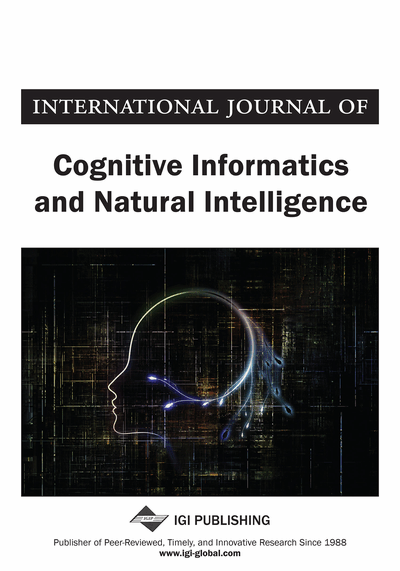# Using the Similarity Measure between Intuitionistic Fuzzy Sets for the Application on Pattern Recognitions

Lixin Fan (Department of Computer Science, Shaoxing University, Shaoxing, China)
DOI: 10.4018/IJCINI.2015040102

## Abstract

The measurement of uncertainty is an important topic for the theories dealing with uncertainty. The definition of similarity measure between two IFSs is one of the most interesting topics in IFSs theory. A similarity measure is defined to compare the information carried by IFSs. Many similarity measures have been proposed. A few of them come from the well-known distance measures. In this work, a new similarity measure between IFSs was proposed by the consideration of the information carried by the membership degree, the non-membership degree, and hesitancy degree in intuitionistic fuzzy sets (IFSs). To demonstrate the efficiency of the proposed similarity measure, various similarity measures between IFSs were compared with the proposed similarity measure between IFSs by numerical examples. The compared results demonstrated that the new similarity measure is reasonable and has stronger discrimination among them. Finally, the similarity measure was applied to pattern recognition and medical diagnosis. Two illustrative examples were provided to show the effectiveness of the pattern recognition and medical diagnosis.
Article Preview
Top

## 1. Introduction

The measurement of uncertainty is an important topic for the theories dealing with uncertainty. The similarity measure, distance measure, entropy in fuzzy set theory, and the relationships among these measures have been extensively studied for their wide applications in image processing, clustering, pattern recognition, case-based reasoning, and many other fields. However, some basic problems still need further investigation. Firstly, a fuzzy soft set is a parameterized family of fuzzy sets on the universe. Different fuzzy soft sets may have different parameter sets. Secondly, in order to cope with different practical problems, it is necessary to find more new similarity measures and entropies and give a deep comparison with the proposals mentioned before. Here the similarity is defined by a mapping between parameter sets. It is an extension of the equality for soft sets but not the similarity measure between soft sets.

The definition of similarity measure between two IFSs is one of the most interesting topics in IFSs theory. A similarity measure is defined to compare the information carried by IFSs. Many similarity measures have been proposed. A few of them come from the well-known distance measures. The first study was carried out by Szmidt and Kacprzyk extending the well-known distances measures, such as the Hamming distance and the Euclidian distance, to IFS environment and comparing them with the approaches used for ordinary fuzzy sets. Therefore, several new distance measures were proposed and applied to pattern recognition. A similarity measure is an important tool of determining the degree of similarity between two objects. Nowadays, many different similarity measures between intuitionistic fuzzy sets (IFSs) have been proposed since Atanassov [Atanassov, 1986] extended fuzzy sets [Zadeh, 1965] to IFSs, which provide a flexible mathematical framework to cope with, besides the presence of vagueness, the hesitancy originating from imperfect or imprecise information. Li and Cheng [D. Li, 2002] discussed some similarity measures on IFSs and proposed a suitable similarity measure between IFSs which is the first one applied to pattern recognition problems. Later, Liang and Shi [Liang, 2003] proposed several similarity measures to differentiate different IFSs and discussed the relationships between these measures. Furthermore, Mitchell [Mitchell, 2003] interpreted IFSs as ensembles of ordered fuzzy sets from a statistical viewpoint to modify Li and Cheng’s measures. Then, Hung and Yang [Hung, 2004] proposed another method to calculate the distance between IFSs based on the Hausdorff distance and used it to generate several similarity measures between IFSs that are suited to be used in linguistic variables. Szmidt and Kacprzyk [Szmidt, 2005] introduced the Hamming distance between IFSs based on the extension of the Hamming distance on fuzzy sets and proposed a similarity measure between IFSs based on the distance and its application in group decision making. Also, Liu [Liu, 2005] proposed some similarity measures between IFSs and between elements by means of the geometrical representation of an IFS and applied the measure methods in pattern recognition. Furthermore, Hung and Yang [Hung, 2007] proposed the similarity measures of intuitionistic fuzzy sets based on Lp metric. On the other hand, Ye [Ye, 2011] proposed a cosine similarity measure of IFSs based on the cosine similarity measure (angular coefficient) in vector space and applied it to pattern recognition and medical diagnosis. However, some similarity measures cannot carry out our comparison and recognition in some cases. This will get the decision maker into trouble in practical applications. Therefore, in this paper we propose a new similarity measure of IFSs. Then, comparing the proposed similarity measure with the existing similarity measures, we find that the similarity measure proposed in this paper is more reasonable than some similarity measures. Finally, the similarity measure is applied to pattern recognition and medical diagnosis.

## Complete Article List

Search this Journal:
Reset
Open Access Articles: Forthcoming
Volume 14: 4 Issues (2020): 1 Released, 3 Forthcoming
Volume 13: 4 Issues (2019)
Volume 12: 4 Issues (2018)
Volume 11: 4 Issues (2017)
Volume 10: 4 Issues (2016)
Volume 9: 4 Issues (2015)
Volume 8: 4 Issues (2014)
Volume 7: 4 Issues (2013)
Volume 6: 4 Issues (2012)
Volume 5: 4 Issues (2011)
Volume 4: 4 Issues (2010)
Volume 3: 4 Issues (2009)
Volume 2: 4 Issues (2008)
Volume 1: 4 Issues (2007)
View Complete Journal Contents Listing